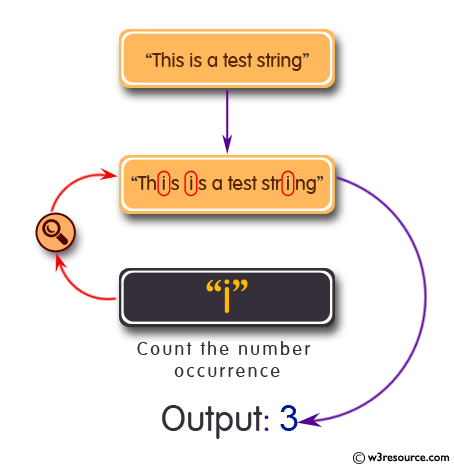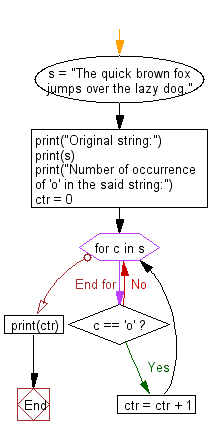﻿ Python: Count the number of occurrence of a specific character in a string - w3resource# Python: Count the number of occurrence of a specific character in a string

## Python Basic: Exercise-84 with Solution

Write a Python program to count the number of occurrence of a specific character in a string.

Pictorial Presentation:Sample Solution:

Using count() function:

Python Code:

``````s = "The quick brown fox jumps over the lazy dog."
print("Original string:")
print(s)
print("Number of occurrence of 'o' in the said string:")
print(s.count("o"))
```
```

Sample Output:

```Original string:
The quick brown fox jumps over the lazy dog.
Number of occurrence of 'o' in the said string:
4
```

## Visualize Python code execution:

The following tool visualize what the computer is doing step-by-step as it executes the said program:

Using loop:

Python Code:

``````s = "The quick brown fox jumps over the lazy dog."
print("Original string:")
print(s)
print("Number of occurrence of 'o' in the said string:")
ctr = 0
for c in s:
if c == 'o':
ctr = ctr + 1
print(ctr)
```
```

Sample Output:

```Original string:
The quick brown fox jumps over the lazy dog.
Number of occurrence of 'o' in the said string:
4
```

Flowchart:## Visualize Python code execution:

The following tool visualize what the computer is doing step-by-step as it executes the said program:

Using collections.Counter():

Python Code:

``````from collections import Counter
s = "The quick brown fox jumps over the lazy dog."
print("Original string:")
print(s)
print("Number of occurrence of 'o' in the said string:")
ctr = Counter(s)
print (str(ctr['o']))
```
```

Sample Output:

```Original string:
The quick brown fox jumps over the lazy dog.
Number of occurrence of 'o' in the said string:
4
```

## Visualize Python code execution:

The following tool visualize what the computer is doing step-by-step as it executes the said program:

Using Lambda functions:

Python Code:

``````s = "The quick brown fox jumps over the lazy dog."
print("Original string:")
print(s)
print("Number of occurrence of 'o' in the said string:")
ctr = sum(map(lambda x : 1 if 'o' in x else 0, s))
print(ctr)
```
```

Sample Output:

```Original string:
The quick brown fox jumps over the lazy dog.
Number of occurrence of 'o' in the said string:
4
```

## Visualize Python code execution:

The following tool visualize what the computer is doing step-by-step as it executes the said program:

Using Regular Expressions:

Python Code:

``````import re
s = "The quick brown fox jumps over the lazy dog."
print("Original string:")
print(s)
print("Number of occurrence of 'o' in the said string:")
ctr = len(re.findall("o", s))
print(ctr)
```
```

Sample Output:

```Original string:
The quick brown fox jumps over the lazy dog.
Number of occurrence of 'o' in the said string:
4
```

## Visualize Python code execution:

The following tool visualize what the computer is doing step-by-step as it executes the said program:

Python Code Editor:

Have another way to solve this solution? Contribute your code (and comments) through Disqus.

What is the difficulty level of this exercise?

Test your Python skills with w3resource's quiz

﻿

## Python: Tips of the Day

Try-catch-else construct:

```try:
foo()
except Exception:
print("Exception occured")
else:
print("Exception didnt occur")
finally:
print("Always gets here")
```